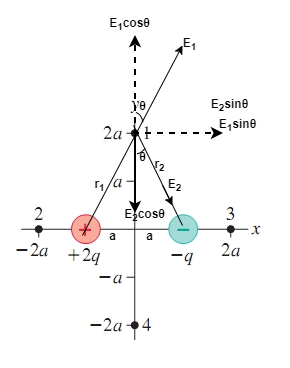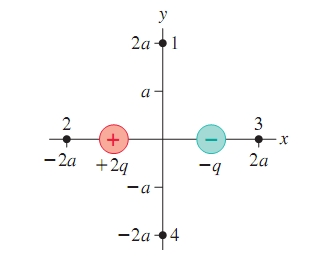# Problem: Charges -q and +2q in the figure are located at x=+-a.  Determine the electric field at points 1 to 4. Write each field in component form.

###### FREE Expert Solution

The electric field due to point charge at distance r:

$\overline{){\mathbf{E}}{\mathbf{=}}\frac{\mathbf{k}\mathbf{q}}{{\mathbf{r}}^{\mathbf{2}}}}$, where q is the charge, r is the distance from the point to the charge, and k is the electrostatic constant.

k is also expressed as:

$\overline{){\mathbf{k}}{\mathbf{=}}\frac{\mathbf{1}}{\mathbf{4}{\mathbf{\pi \epsilon }}_{\mathbf{0}}}}$

Therefore, we have the electric field written as:

$\overline{){\mathbf{E}}{\mathbf{=}}\frac{\mathbf{1}}{\mathbf{4}{\mathbf{\pi \epsilon }}_{\mathbf{0}}}\frac{\mathbf{q}}{\mathbf{r}}}$

We'll consider the following figure.At point 1

The distance between charge 2q and point 1 is:

$\begin{array}{rcl}{{\mathbf{r}}_{\mathbf{1}}}^{\mathbf{2}}& \mathbf{=}& {\mathbf{\left(}\mathbf{-}\mathbf{a}\mathbf{\right)}}^{\mathbf{2}}\mathbf{+}{\mathbf{\left(}\mathbf{2}\mathbf{a}\mathbf{\right)}}^{\mathbf{2}}\\ & \mathbf{=}& {\mathbf{a}}^{\mathbf{2}}\mathbf{+}\mathbf{4}{\mathbf{a}}^{\mathbf{2}}\end{array}$

r12 = 5a2

The distance between charge, -q and point 1 is:

$\begin{array}{rcl}{{\mathbf{r}}_{\mathbf{2}}}^{\mathbf{2}}& \mathbf{=}& {\mathbf{a}}^{\mathbf{2}}\mathbf{+}{\mathbf{\left(}\mathbf{2}\mathbf{a}\mathbf{\right)}}^{\mathbf{2}}\\ & \mathbf{=}& {\mathbf{a}}^{\mathbf{2}}\mathbf{+}\mathbf{4}{\mathbf{a}}^{\mathbf{2}}\end{array}$

r22 = 5a2

From our figure:

$\begin{array}{rcl}\mathbf{s}\mathbf{i}\mathbf{n}\mathbf{\theta }& \mathbf{=}& \frac{\mathbf{a}}{{\mathbf{r}}_{\mathbf{2}}}\\ & \mathbf{=}& \frac{\mathbf{a}}{\sqrt{\mathbf{5}{\mathbf{a}}^{\mathbf{2}}}}\\ & \mathbf{=}& \frac{\overline{)\mathbf{a}}}{\overline{)\mathbf{a}}\sqrt{\mathbf{5}}}\end{array}$

sinθ = 1/sqrt(5)

Also,

$\begin{array}{rcl}\mathbf{c}\mathbf{o}\mathbf{s}\mathbf{\theta }& \mathbf{=}& \frac{\mathbf{2}\mathbf{a}}{{\mathbf{r}}_{\mathbf{2}}}\\ & \mathbf{=}& \frac{\mathbf{2}\mathbf{a}}{\sqrt{\mathbf{5}{\mathbf{a}}^{\mathbf{2}}}}\\ & \mathbf{=}& \frac{\mathbf{2}\overline{)\mathbf{a}}}{\overline{)\mathbf{a}}\sqrt{\mathbf{5}}}\end{array}$

cosθ = 2/sqrt(5)

The electric field at point 1 due to charge, +2q is:

90% (346 ratings)###### Problem DetailsCharges -q and +2q in the figure are located at x=+-a.  Determine the electric field at points 1 to 4. Write each field in component form.

Frequently Asked Questions

What scientific concept do you need to know in order to solve this problem?

Our tutors have indicated that to solve this problem you will need to apply the Electric Field concept. You can view video lessons to learn Electric Field. Or if you need more Electric Field practice, you can also practice Electric Field practice problems.